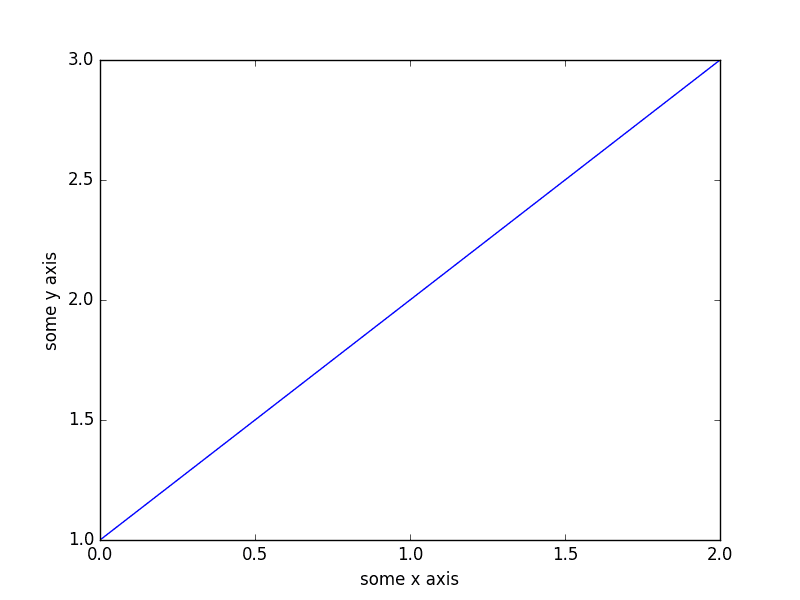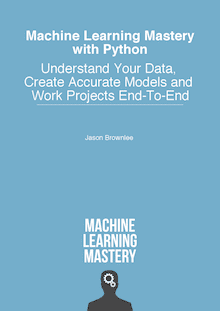Crash Course in Python for Machine Learning Developers

Last Updated on August 21, 2019

You do not need to be a Python developer to get started using the Python ecosystem for machine learning.

As a developer who already knows how to program in one or more programming languages, you are able to pick up a new language like Python very quickly. You just need to know a few properties of the language to transfer what you already know to the new language.

In this post, you will get a crash course in Python and the core libraries needed for machine learning. Namely: NumPy, MatPlotLib and Pandas.

This will be just enough information to help you read and understand code Python code examples for machine learning and start developing your own scripts. If you already know a little Python, this post will be a friendly reminder for you.

Kick-start your project with my new book Machine Learning Mastery With Python, including step-by-step tutorials and the Python source code files for all examples.

Let’s get started.

• Update Mar/2017: Updated all print statements to work with Python 2 and Python 3.Crash Course in Python for Machine Learning Developers
Photo by John Clouston, some rights reserved.

Python Crash Course

When getting started in Python you need to know a few key details about the language syntax to be able to read and understand Python code. This includes:

• Assignment
• Flow Control
• Data Structures
• Functions

We will cover each of these topics in turn with small standalone examples that you can type and run.

Remember, whitespace has meaning in Python.

Need help with Machine Learning in Python?

Take my free 2-week email course and discover data prep, algorithms and more (with code).

Click to sign-up now and also get a free PDF Ebook version of the course.

Assignment

As a programmer, assignment and types should not be surprising to you.

Strings

Running the example prints:

Numbers

Running the example prints:

Boolean

Running the example prints:

Multiple Assignment

Running the example prints:

No Value

Running the example prints:

Flow Control

There are three main types of flow control that you need to learn: If-Then-Else conditions, For-Loops and While-Loops.

If-Then-Else Condition Example

Running this example prints:

For-Loop Example

Running this example prints:

While-Loop Example

Running this example prints:

Data Structures

There are three data structures in Python that you will find the most used and useful. They are tuples, lists and dictionaries.

Tuple Example

Tuples are read-only collections of items.

Running the example prints:

List Example

Lists use the square bracket notation and can be index using array notation.

Running the example prints:

Dictionary Example

Dictionaries are mappings of names to values, like a map. Note the use of the curly bracket notation.

Running the example prints:

Functions

The biggest gotcha with Python is the whitespace. Ensure that you have an empty new line after indented code.

The example below defines a new function to calculate the sum of two values and calls the function with two arguments.

Running the example prints:

NumPy Crash Course

NumPy provides the foundation data structures and operations for SciPy. These are arrays (ndarrays) that are efficient to define and manipulate.

Create Array

Running the example prints:

Access Data

Array notation and ranges can be used to efficiently access data in a NumPy array.

Running the example prints:

Arithmetic

NumPy arrays can be used directly in arithmetic.

Running the example prints:

There is a lot more to NumPy arrays but these examples give you a flavor of the efficiencies they provide when working with lots of numerical data.

Matplotlib Crash Course

Matplotlib can be used for creating plots and charts.

The library is generally used as follows:

1. Call a plotting function with some data (e.g. plot()).
2. Call many functions to setup the properties of the plot (e.g. labels and colors).
3. Make the plot visible (e.g. show()).

Line Plot

The example below creates a simple line plot from one-dimensional data.

Running the example produces:Simple Line Plot in Matplotlib

Scatter Plot

Below is a simple example of creating a scatter plot from two-dimensional data.

Running the example produces:Simple Scatter Plot in Matplotlib

There are many more plot types and many more properties that can be set on a plot to configure it.

Pandas Crash Course

Pandas provides data structures and functionality to quickly manipulate and analyze data. The key to understanding Pandas for machine learning is understanding the Series and DataFrame data structures.

Series

A series is a one-dimensional array where the rows and columns can be labeled.

Running the example prints:

You can access the data in a series like a NumPy array and like dictionary, for example:

Running the example prints:

DataFrame

A data frame is a multi-dimensional array where the rows and the columns can be labeled.

Running the example prints:

Data can be index using column names.

Running the example prints:

Summary

You have covered a lot of ground in this post. You discovered basic syntax and usage of Python and four key Python libraries used for machine learning:

• NumPy
• Matplotlib
• Pandas

You now know enough syntax and usage information to read and understand Python code for machine learning and to start creating your own scripts.

Discover Fast Machine Learning in Python!Develop Your Own Models in Minutes

...with just a few lines of scikit-learn code

Learn how in my new Ebook:
Machine Learning Mastery With Python

Covers self-study tutorials and end-to-end projects like:

24 Responses to Crash Course in Python for Machine Learning Developers

1.MouChenghao September 9, 2016 at 9:51 pm #

I guess there is a wrong output in the last example, where the correct result should be like this:

one column: a 1
b 4
Name: one, dtype: int64
one column: a 1
b 4
Name: one, dtype: int64

•Jason Brownlee September 10, 2016 at 7:14 am #

You are right, fixed. I have edited it for readability and broken the output. Sorry.

2.Surya April 5, 2017 at 5:38 pm #

Hi Jason, on first string example printing data would be “h” and not 4.

•Jason Brownlee April 9, 2017 at 2:33 pm #

Thanks Surya.

3.anon September 22, 2017 at 6:43 am #

Great post! Quick fix, the if-else-if is a bit wonky, ‘That is too fast’ will never print.

•Jason Brownlee September 23, 2017 at 5:32 am #

Thanks.

4.Ahmed July 14, 2018 at 6:33 pm #

How can I go to the next line without executing the code ?

>>> value = 99
>>> if value >= 99:
… print (‘That is fast’)
File “”, line 2
print (‘That is fast’)
^
IndentationError: expected an indented block
>>>

•Jason Brownlee July 15, 2018 at 6:10 am #

Sounds like you need to indent the Python code correctly.

https://machinelearningmastery.com/faq/single-faq/how-do-i-copy-code-from-a-tutorial

•Ahmed July 15, 2018 at 4:30 pm #

Actully I was doing that, but I thought that typing the code would give me better understanding of it rather than just copy it ( what do you think ?)
so I’ve decided to type them but I don’t now how to go to next line without running the code

5.Ahmed July 17, 2018 at 2:16 pm #

Thanks a lot , very clear explanation

•Jason Brownlee July 17, 2018 at 2:32 pm #

Thanks.

6.Carlos Morente August 4, 2018 at 8:24 pm #

Hi Jason!

Just writing you to say that you made a little mistake in the “running the example prints” for Strings, the first output should be ‘h’ and not ‘4’.

•Jason Brownlee August 5, 2018 at 5:28 am #

Thanks, fixed.

7.Clint Laskowski December 11, 2018 at 10:58 pm #

Really nice (and quick) introduction to Python, NumPy, Matplotlib, and Pandas. Looking into your book now 🙂

•Jason Brownlee December 12, 2018 at 5:54 am #

Thanks Clint.

8.laz65 December 6, 2019 at 10:10 am #

many thanks jason 😉

•Jason Brownlee December 6, 2019 at 1:39 pm #

You’re welcome.

9.Robbie February 4, 2020 at 7:24 pm #

Hi Jason,

In the “IF-Then-Else Condition Example”, i would expect by changing the value to 300 the code should print ‘That is too fast’. However, the output is ‘That is fast’. Can you please explain?

#Output
That is fast

•Jason Brownlee February 5, 2020 at 8:05 am #

The first condition was executed first and passed.

You can re-order the if-statements or use a switch/case statement.

10.MoJeeza February 6, 2020 at 7:26 am #

This should work..

•Jason Brownlee February 6, 2020 at 8:34 am #

Nice work!

11.MoJeeza February 6, 2020 at 7:29 am #

Somehow the indentation was lost during paste

•Jason Brownlee February 6, 2020 at 8:34 am #

I fixed it for you. by adding PRE tags.